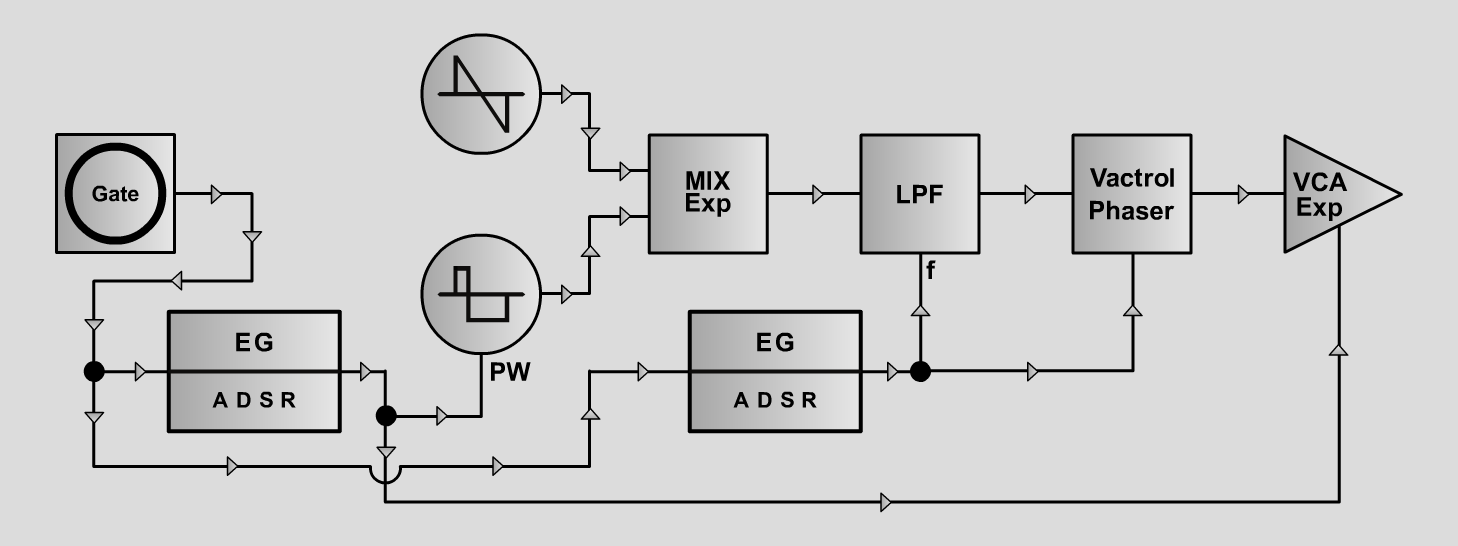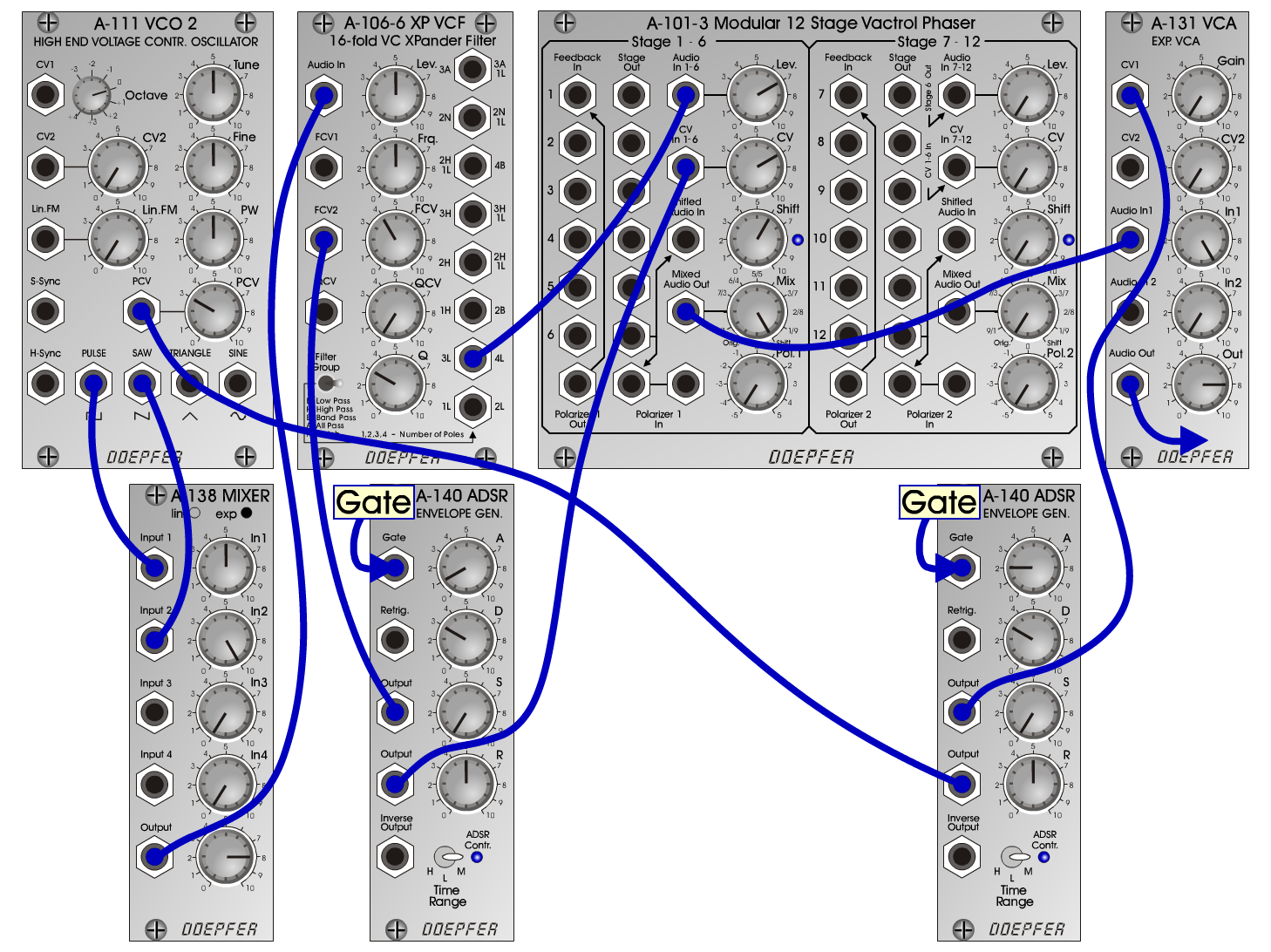S h o r t yS y n t hD o e p f e r A - 100 Connections: Settings: Shorty1 A-111 (Pulse) <=> A-138exp (Input 1)  A-111 (Saw) <=> A-138exp (Input 2)  A-138exp (Output) <=> A-106-6 (Audio In)  A-106-6 (4L) <=> A-101-3 (Audio In 1-6)  A-101-3 (Mixed Audio Out) <=> A-131 (Audio In)  A-140/1 (Output 1) <=> A-106-6 (FCV2)  A-140/1 (Output 2) <=> A-101-3 (CV In 1-6)  A-140/2 (Output 1) <=> A-131 (CV1)  A-140/2 (Output 2) <=> A-111 (PCV) A-111 (PW = 5, PCV = 3)  A-138exp (In 1 = 5, In 2 = 10, Out = 8)  A-106-6 (Lev = 5, Frq = 5, FCV = 4, Q = 3)  A-101-3 (Lev = 7, CV = 7, Shift = 6, Mix = 10, Pol 1 = 0)  A-140/1 (A = 1, D = 3, S = 0, R = 5, Range = M)  A-140/2 (A = 2, D = 3, S = 0, R = 5, Range = M)  A-131 (Gain = 0, Audio In 1 = 10, Audio Out = 8) Shorty2 A-111 (PW = 5, PCV = 3)  A-138exp (In 1 = 10, In 2 = 5, Out = 8)  A-106-6 (Lev = 5, Frq = 5.5, FCV = 2.1, Q = 3)  A-101-3 (Lev = 7, CV = 7, Shift = 6, Mix = 10, Pol 1 = 0)  A-140/1 (A = 3, D = 3, S = 0, R = 5, Range = M)  A-140/2 (A = 2, D = 3, S = 0, R = 5, Range = M)  A-131 (Gain = 0, Audio In 1 = 10, Audio Out = 8) Shorty3 A-111 (PW = 5, PCV = 3)  A-138exp (In 1 = 10, In 2 = 5, Out = 8)  A-106-6 (Lev = 5, Frq = 5, FCV = 2.1, Q = 3)  A-101-3 (Lev = 7, CV = 7, Shift = 6, Mix = 10, Pol 1 = 0)  A-140/1 (A = 0, D = 3, S = 0, R = 5, Range = M)  A-140/2 (A = 0, D = 3, S = 0, R = 5, Range = M)  A-131 (Gain = 0, Audio In 1 = 10, Audio Out = 8) Josef MuellerSound samples Bach Praeludium (BWV 847) Shorty 1 (131 Hz) Shorty 1 (262 Hz) Shorty 1 (523 Hz) Shorty 2 (131 Hz) Shorty 2 (262 Hz) Shorty 2 (523 Hz) Shorty 3 (131 Hz) Shorty 3 (262 Hz) Shorty 3 (523 Hz)